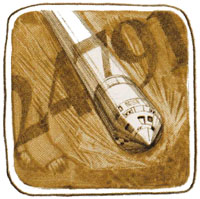Section 12: Subroutines190
Keystrokes Display
ALPHA RATE? ALPHA
 05TRATE?

PROMPT ( Σ+
 06 PROMPT

ALPHA TIME? ALPHA
 07TTIME?

PROMPT ( Σ+
 08 PROMPT

×
 09 *

g RTN
 10 RTN
End of subroutine A.
g LBL
ALPHA B ALPHA
 11 LBL B
Local label B.
ALPHA DISTANCE? ALPHA
 12TDISTANCE?

PROMPT ( Σ+
 13 PROMPT

ALPHA TIME? ALPHA
 14TTIME?

PROMPT ( Σ+
 15 PROMPT

÷
 16 /

g RTN
 17 RTN
End of subroutine B.
g LBL
ALPHA C ALPHA
 18 LBL C
Local label C.
ALPHA
DISTANCE? ALPHA
 19TDISTANCE?

PROMPT ( Σ+
 20 PROMPT

ALPHA RATE? ALPHA
 21TRATE?

PROMPT ( Σ+
 22 PROMPT

÷
 23 /

g GTO · ·
 00 REG 33
End of subroutine C.
Now run the program to solve the following problem:
On may 26, 1969 the Command and Service Module of Apollo X carried U.S. astronauts Stafford, Cernan, and Youngh at a rate of 24,791 miles per hour (the fastest speed at which any human has traveled). How far would the module travel in 2.5 hours?
 D = R T = 24,791 × 2.4